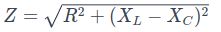# Impedance in Electricity: Definition, Components and ExamplesElectricity is an essential part of our daily lives. From turning on a light to charging our electronic devices, we rely on electrical current to perform a wide variety of tasks.

However, for electricity to flow efficiently and safely, it is essential to understand one key concept: electrical impedance.

In this article, we will explain in detail what impedance is in electricity, how it affects electrical circuits and why it is important in the modern world.

## What Is Impedance?

Impedance in electricity can be defined as the dynamic resistance that an electrical circuit presents to alternating current (AC). Unlike electrical resistance, which applies to direct current (DC) circuits, impedance is a measure of the opposition a circuit offers to fluctuating current in an AC circuit.

In other words, it is the resistance that varies with the frequency of the electric current.

Impedance is usually represented by the letter "Z" and is measured in ohms (Ω), just like electrical resistance. However, unlike resistance, which is constant in a DC circuit, impedance can vary significantly with the frequency of the alternating current. This is due to the influence of components such as inductances and capacitances in the circuit.

## Components of Impedance

The impedance of an electrical circuit is made up of three main components: resistance (R), inductance (L), and capacitance (c).

Each of these components contributes uniquely to the total impedance of the circuit.

### Resistance (R)

This component of impedance is analogous to electrical resistance in DC circuits. It represents the opposition to the flow of electrical current due to the friction that electricity experiences as it passes through conductors and other circuit components.

### Inductance (L)

Inductance refers to the ability of a circuit to oppose changes in electrical current. When alternating current flows through a coil, it induces a magnetic field that opposes changes in current.

Inductance contributes to impedance increasing with the frequency of the current.

### Capacitance (C)

Capacitance refers to the ability of a circuit to store energy in an electric field.

When alternating current flows through a capacitor, it stores and releases energy in a cyclical manner. Capacitance also contributes to impedance and decreases with current frequency.

## Examples of Impedance

Here I show you some examples of impedance in different contexts and applications:

### Speakers in an Audio SystemIn an audio system, speakers have a nominal impedance (for example, 8 ohms). For optimal performance, it is important that the speaker impedance matches the output impedance of the amplifier. If they do not match, there may be a loss of power and sound quality.

### Electrical Energy Transmission

In high voltage electrical power transmission systems, cables and transmission lines have an inherent impedance that affects transmission efficiency. Impedance calculation is used to minimize energy losses as heat and to optimize energy transfer.

Antennas used in radio and communications systems have a characteristic impedance that must match the impedance of the transmitting and receiving devices. This is done to maximize the transfer of radio frequency signals and minimize signal reflections.

### Radio Frequency (RF) Electronic Circuits

In RF circuits, impedance is essential for the design of amplifiers, filters, and transmission lines. Components and circuits are carefully designed to match the impedance of other components in the system and to accommodate RF signals.

### Filters and Noise Suppression Circuits

In applications where electrical noise is sought to be filtered or suppressed, such as in high-fidelity audio equipment, circuits with specific impedances are used to achieve the desired effects.

## Impedance and Frequency

A key aspect of impedance is its dependence on the frequency of the alternating current. As frequency increases, inductance generally has a more significant effect on impedance, resulting in an increase in impedance. On the other hand, as frequency decreases, capacitance tends to have a more pronounced effect, decreasing impedance.

This phenomenon is fundamental in radio and communications electronics, where the impedance of antennas and radio circuits must be carefully tuned to maximize energy transfer at a particular frequency.

## Impedance Calculation

Calculating the impedance of an electrical circuit can be a complex task due to the interaction of the R, L and C components.

However, in simple circuits, it can be calculated using Ohm's law for impedance, which is similar to Ohm's law for electrical resistance, but takes complex impedance into account:Where:

• Z is the total impedance.

• R is the resistance in ohms.

• XL is the inductive reactance in ohms.

• XC is the capacitive reactance in ohms.

Inductive reactance (XL) and capacitive reactance (XC) are complex values ​​that depend on the frequency of the alternating current.

Author:

Published: March 9, 2020
Last review: October 6, 2023